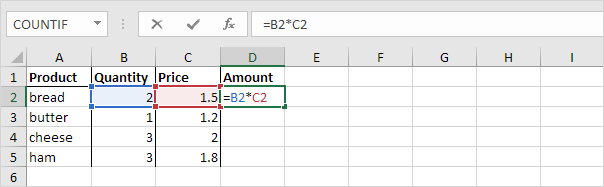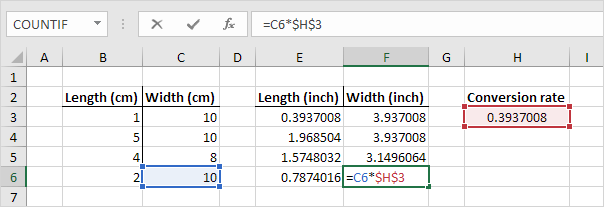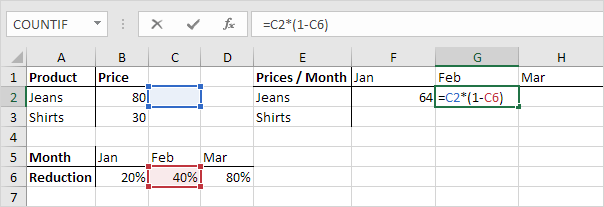Cell References in Excel (In Easy Steps)

0
99

Cell references in Excel

Relative Reference

By default, Excel uses relative reference. See the formula in cell D2 below. Cell D2 references (points to) cell B2 and cell C2. Both references are relative.1. Select cell D2, click on the lower right corner of cell D2 and drag it down to cell D5.Absolute Reference

1. To create an absolute reference to cell H3, place a \$ symbol in front of the column letter and row number of cell H3 (\$H\$3) in the formula of cell E3.2. Now we can quickly drag this formula to the other cells.Mixed Reference

1. See the formula in cell F2 below.2. We want to copy this formula to the other cells quickly. Drag cell F2 across one cell, and look at the formula in cell G2.Do you see what happens? The reference to the price should be a fixed reference to column B. Solution: place a \$ symbol in front of the column letter of cell B2 (\$B2) in the formula of cell F2. In a similar way, when we drag cell F2 down, the reference to the reduction should be a fixed reference to row 6. Solution: place a \$ symbol in front of the row number of cell B6 (B\$6) in the formula of cell F2.